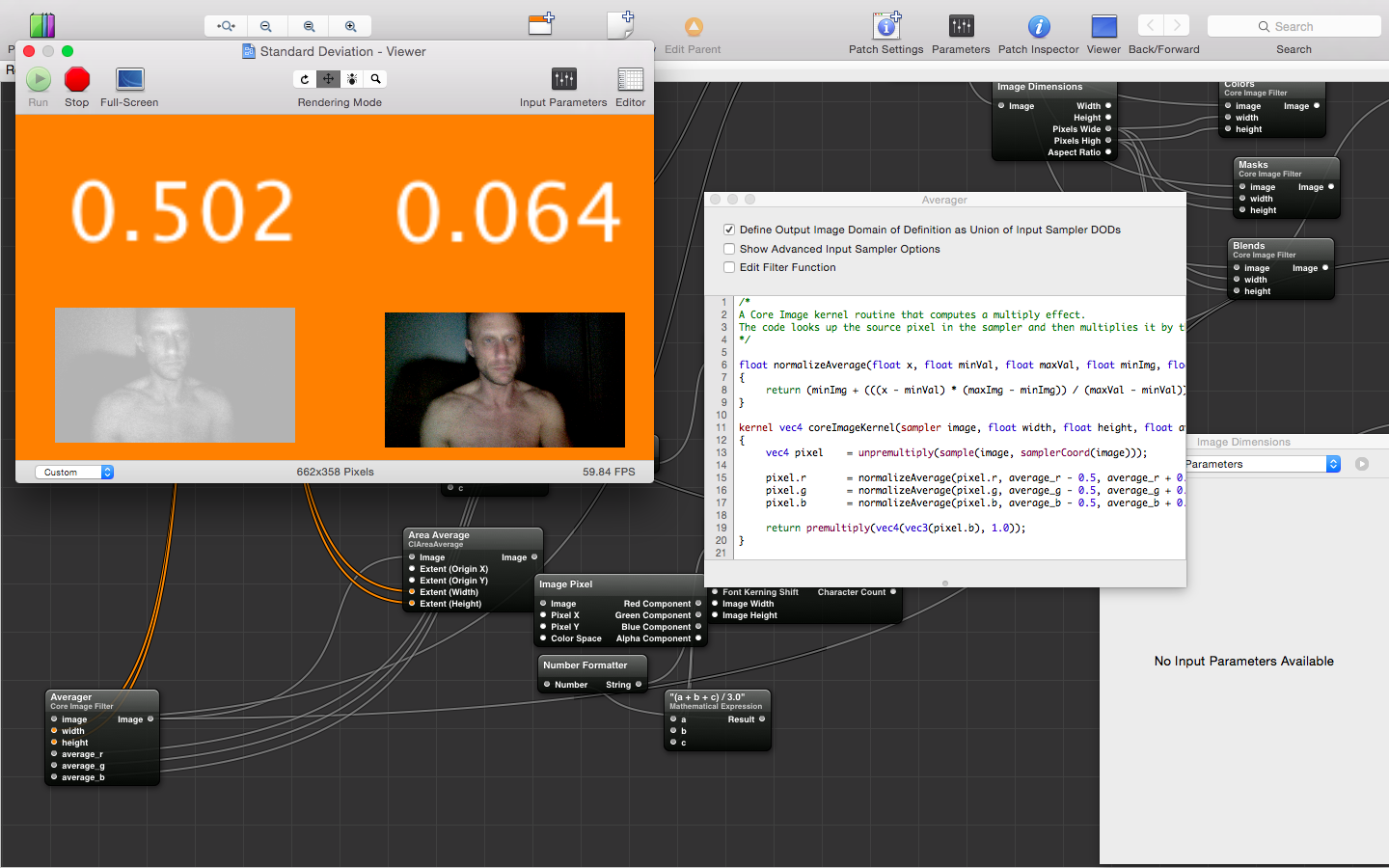## Friday, April 3, 2015

### TECHNOLOGY | A loaf of evil, one slice at-a-time

Rough approximation of the next post to the blog:

Final Gaussian Curve prototype
Early adopters of the series of decloaking image filters for iPhone can try out the Gaussian Curve filter in Quartz Composer right now; those who want (or need) an iPhone version may request it.

The filter averages two Gaussian curves, allowing for high-pass, low-pass, band-pass, band-stop and unsharp mask filtering:Granular confinement of any portion of the visible (and invisible) spectrum is now at the hands of anyone who understands curves and their relationship to image processing
This is not a user-friendly setup, by any means; an understanding of these curves and how they apply to image processing is essential.

High-pass filtering
A test of the first iteration of high-pass frequency scanning filter for the iPhone camera, which allows you to isolate the image to a specific range of values and frequency, and the alter the tonal (soon, radiance) map, in order to focus on and enhance specific portions:

The purpose is to focus on things that can only be seen when all other things are blocked out, and to enhance just those things using standard image enhancement methods. Essentially, it slices the a loaf of bread that is your image, and allows you to examine each slice at a time:

float f(float x, float peak, float midpoint, float width, float y)
{
const float pi = 4.0 * atan(1.0);
//float e_p = pow(pow((1.0 + (1.0 / x)), x), x);
//float e_n = pow(pow((1.0 + (1.0 / x)), x), -x);
float a = 1.0 / (peak * sqrt(2.0 * pi));
return (a * exp(-(pow((x - midpoint), 2.0)) / (2.0 * pow(width, 2.0)))) + y;
}

float g(float x, float peak, float midpoint, float width, float y)
{
const float pi = 4.0 * atan(1.0);
//float e_p = pow(pow((1.0 + (1.0 / x)), x), x);
//float e_n = pow(pow((1.0 + (1.0 / x)), x), -x);
float a = 1.0 / (peak * sqrt(2.0 * pi));
return 1.0 - (a * exp(-(pow((x - midpoint), 2.0)) / (2.0 * pow(width, 2.0)))) + y;
}

float i(float f, float g)
{
return (f - g) / 2.0;
}

float j(float x, float l, float u)
{
return (x - l) * ((1.0 - 1e-10) / (u - l)) + 1e-10;
}

kernel vec4 coreImageKernel(sampler image, float w, float h, float peak, float peaky, float midpoint, float midpointy, float width, float widthy, float y, float z)
{
vec4 pixel = sample(image, samplerCoord(image));

float alpha = j(f(destCoord().x / w, peak, midpoint, width, y), peak, peaky);
float alphay = j(g(destCoord().x / w, peaky, midpointy, widthy, z), peak, peaky);
vec4 gr = vec4(i(alpha, alphay));
float height_scale   = float(gr) * h;
float dist = abs(destCoord().y - height_scale);

float s = f(pow(max(pixel.r, max(pixel.g, pixel.b)), 1.0 / 3.0), peak, midpoint, width, y);
float t = g(pow(max(pixel.r, max(pixel.g, pixel.b)), 1.0 / 3.0), peaky, midpointy, widthy, z);
vec4 x = vec4(i(s, t));
x = premultiply((dist < 1.0/100.0 * h) ? gr : x);
x = (destCoord().y < 10.0/100.0 * h) ? gr : x;

return x;
}Damage caused by demonic weapons fire to the eyes is easily detectable by screening with this new high-pass/high-contrast, which works similar to the eye-dye optometrists use
Why is this particular filter necessary? As discussed in Comparing demonic activity in light and shadow and Demonic Feng Shui, demons and related demonic activity happens on the fringe between light and dark, and those areas are not any camera's forte. This filter allows you to isolate such twilight zones on the display, and then dramatically increase contrast, so that things inside them appear as if they were illuminated by strong sunlight.

Mean averaging
Instead of using the blue channel-enhancement procedure described in [link], you can normalize (or stretch) values in each color component using its image-wide average to adjust the minimum and maximum values [see Feature Scaling on Wikipedia.org for more information on the formula]:

/*
A Core Image kernel routine that averages the pixel values of an image to 0.5
The code looks up the source pixel in the sampler and then normalizes its value using the average as the minimum or maximum, depending on whether its value is greater or less than the average.
*/

float normalizeAverage(float x, float minVal, float maxVal, float minImg, float maxImg)
{
return (minImg + (((x - minVal) * (maxImg - minImg)) / (maxVal - minVal)));
}

kernel vec4 coreImageKernel(sampler image, float width, float height, float average_r, float average_g, float average_b)
{
vec4 pixel = unpremultiply(sample(image, samplerCoord(image)));

pixel.r = normalizeAverage(pixel.r, average_r - 0.5, average_r + 0.5, 0.0, 1.0);
pixel.g = normalizeAverage(pixel.g, average_g - 0.5, average_g + 0.5, 0.0, 1.0);
pixel.b = normalizeAverage(pixel.b, average_b - 0.5, average_b + 0.5, 0.0, 1.0);

return premultiply(vec4(vec3(pixel.r), 1.0));
}

text:The average pixel value of the value-adjusted image (left), at the midpoint in the (0,1) range
text.